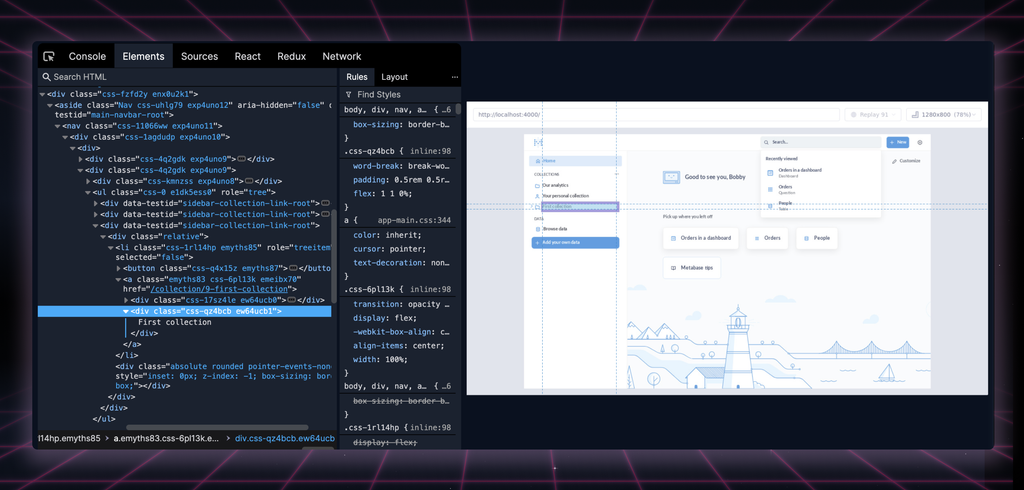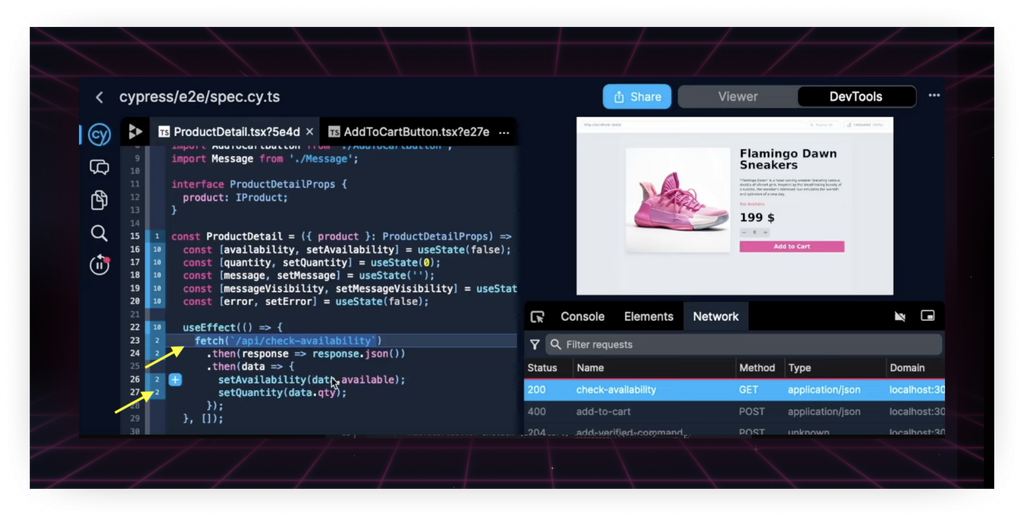# Random tangent on program stateJosh Morrow
📌
I had high hopes for this, but I think it’s just not the right set of examples to use. I think the idea that traversing execution space using snapshots is fast but verbose, and traversing using program execution is compact but computationally expensive is a powerful way of explaining it, but it needs a better set of examples to really get the point across, I think.
Before we move on, let’s imagine the program as a series of discrete states. The longer the recording, the more states it contains. This series of states can be described as a timeline, but a timeline is kind of just a one-dimensional space. We can traverse that space by applying program transformations one on top of the other (following the program’s code) or we can apply the summation of all of the changes of a particular set of computations. Let’s take the following program for instance:
```javascript```export function recaman(): () => number {
const seen = new Set<number>();
let current = 0;
let step = 0;
return () => {
const next = current - step;
if (next < 0 || seen.has(next)) {
current += step + 1;
step++;
} else {
current = next;
}
return current;
};
}

function main() {
const sequence = recaman();
for (let i = 0; i < 10; i++) {
console.log(sequence());
}
}

main();``````
This script will output the first ten numbers of the recaman sequence. At each step of the sequence, the program’s state is changing. Notice: it’s completely deterministic. We could create a perfect recording of this program with basically zero outside input. What if, rather than something completely deterministic, we had a somewhat random recaman sequence?
```javascript```export function recaman(): () => number {
const seen = new Set<number>();
let current = 0;
let step = 0;
return () => {
const next = current - step;
if (Math.random() > 0.5) {
current += step + 1;
step++;
} else {
current = next;
}
return current;
};
}

function main() {
const sequence = recaman();
for (let i = 0; i < 10; i++) {
console.log(sequence());
}
}

main();``````
Now, we could not predict exactly what the sequence would do next without knowing what `Math.random()` will return. The recording data in this case would contain the pieces of possible non-determinism: the results of calling `Math.random()`, but everything else can be generated by running the program.
Related postsToday we’re excited to share our new Elements Panel. The next time you open a replay and inspect an element you’ll feel the difference.Today, we’re excited to share our progress helping customers debug their flaky tests.